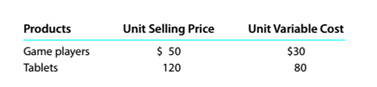Chapter 11, Problem 11.20E

Chapter
Section
Textbook Problem

Sales mix and break-even sales Northwest Technology Inc. manufactures and sells two products, digital game players and computer tablets. The fixed costs are $936,000, and the sales mix is 70% game players and 30% computer tablets. The unit selling price and the unit variable cost for each product are as follows:a.Compute the break-even sales (units) for the overall product, E. b.How many units of each product, game players and tablets, would l>e -sold at the breakeven point? To determine Concept Introduction: Cost Volume Profit (CVP) Analysis: The Cost Volume Profit analysis is the analysis of the relation between cost, volume, and profit of a product. It analyzes the cost and profits at the different level of production, in order to determine the breakeven point and required the level of sales to earn the desired profit. Contribution margin means the margin that is left with the company after recovering variable cost out of revenue earned by selling smart phones. The formula for contribution margin is as follows: Contribution margin = Sales - Variable cost. Similarly contribution margin ratio = Contribution/sales Breakeven Point: The Breakeven point is the level of sales at which the net profit is nil. It can be explained as a situation where the business is generating a sale that is equal to the expenses incurred and hence no profits no loss. Breakeven point in units is calculated with the help of following formula: Breakeven point (units) = Total Fixed Costs(Sales Price Per unit -Variable Cost per unit) Weighted Average Contribution Margin: Weighted Average Contribution Margin is calculated for two products with the help of following formula: Weighted Average Contribution Margin = (Contribution margin of Product A * Weight of product A) + (Contribution margin of Product B * Weight of product B) Requirement-a: To Calculate: The Overall Breakeven Sales unit Explanation The Overall Breakeven point in units is calculated as follows:  Game Players Tablets Overall Unit Selling Price (A)$ 50 $120 Unit Variable Cost (B)$ 30 $80 Unit Contribution Margin (C) =(A-B)$ 20 \$ 4...
To determine

Concept Introduction:

Cost Volume Profit (CVP) Analysis:

The Cost Volume Profit analysis is the analysis of the relation between cost, volume, and profit of a product. It analyzes the cost and profits at the different level of production, in order to determine the breakeven point and required the level of sales to earn the desired profit.

Contribution margin means the margin that is left with the company after recovering variable cost out of revenue earned by selling smart phones. The formula for contribution margin is as follows:

Contribution margin = Sales - Variable cost.

Similarly contribution margin ratio = Contribution/sales

Breakeven Point:

The Breakeven point is the level of sales at which the net profit is nil. It can be explained as a situation where the business is generating a sale that is equal to the expenses incurred and hence no profits no loss. Breakeven point in units is calculated with the help of following formula:

Breakeven point (units) = Total Fixed Costs(Sales Price Per unit -Variable Cost per unit)

Weighted Average Contribution Margin:

Weighted Average Contribution Margin is calculated for two products with the help of following formula:

Weighted Average Contribution Margin = (Contribution margin of Product A * Weight of product A)                                                                       + (Contribution margin of Product B * Weight of product B)

Requirement-b:

To Calculate:

The Breakeven Sales unit for each product

Still sussing out bartleby?

Check out a sample textbook solution.

See a sample solution

The Solution to Your Study Problems

Bartleby provides explanations to thousands of textbook problems written by our experts, many with advanced degrees!

Get Started

Find more solutions based on key concepts